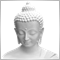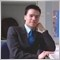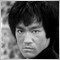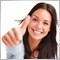• 概述
• 评论 (5)
• 评论 (7)

# Ms Fibonacci

The Ms. Fibonacci is a FREE indicator that automatically draws and adjust new highest and lowest Fibonacci levels on the chart. The most important thing is, draw the Fibonacci levels correctly point to point and mark that price levels.

### Features

• Draw Fibonacci levels and automatically adjust each level.
• Most important Fibo Levels are included (0, 23.6, 38.2, 50.0, 61.8, 100.0, 161.8).
• User friendly parameters.
• Lines, Labels, colors and all other items can customize for a better look.

### Parameters

• C_Fibonacci_Parameters - Fibonacci Parameters.
• Draw a Fibonacci - Activate/ Deactivate, Draw Fibonacci levels on the chart.
• Auto Adjust - Activate/ Deactivate, Automatically adjust Fibonacci High and Low levels.
• Line Style - Fibonacci line style
• Line Width - Fibonacci line width
• Line Color - Fibonacci line color
• Number Of Bars To Search - Draw Fibonacci between last bar and given bar. (Default: 0 = Window First Visible Bar)
• Shift - Shift from last bar.

The Ms. Fibonacci is a FREE version that can be only draws and automatically adjust Fibonacci levels. An unlimited version can be purchased at the following link: Mr. Fibonacci580
2018.12.06 00:47

Cant change colors so it isn't visable with my background12736
2018.06.30 14:09

2 Star (40%) = Undecided

3 Star (60%) = Acceptable

4 Star (80%) = Good

5 Star (100%) = Excellente!5422
2018.02.27 19:45

Good1237
2018.01.17 19:09

perfect484
2017.12.25 10:09